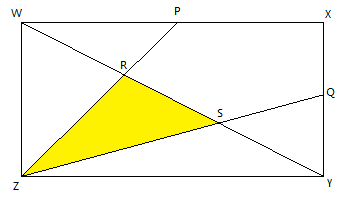# What's C and N?Let $C$ be the answer to this problem and $N$ be the answer to this problem.

Let $WXYZ$ be a nondegenerate rectangle such that $WX = C$ and $XY = N$. Let $P, Q$ be the midpoints of $WX, XY$ respectively. Suppose $WY$ and $ZP$ intersects on $R$, and $WY$ and $ZQ$ intersects on $S$. What is the area of the triangle $RSZ$?

×